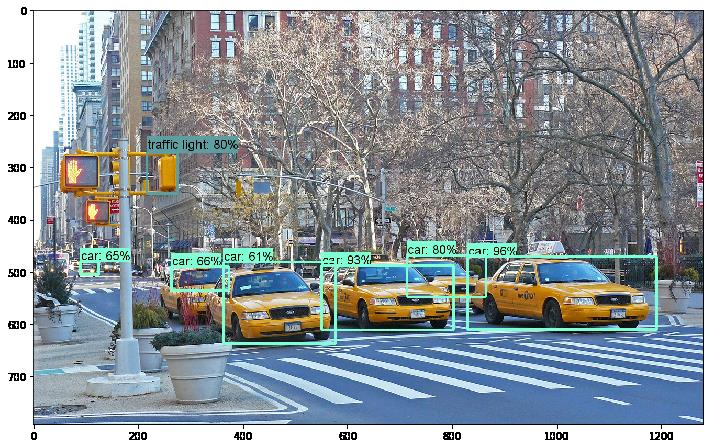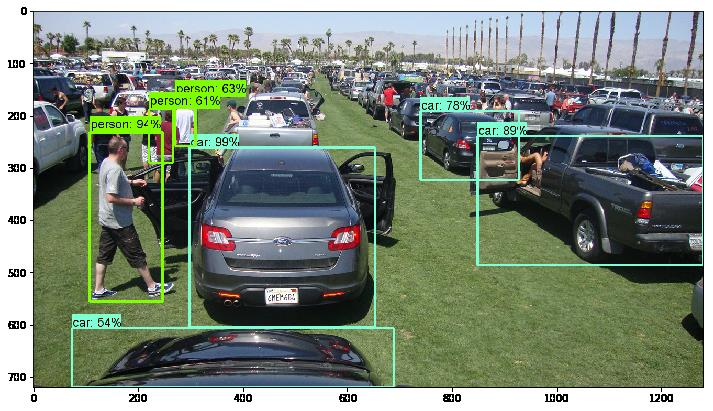Tensorflow Object Detection Tutorial on Images

The tensorflow object detection api is a great tool for performing YOLO object detection. This api comes ready to use with pretrained models which will get you detecting objects in images or videos in no time.

The object detection api does not come standard with the tensorflow installation. You must go through a series of steps in order to install it. I have outlined the steps to install the object detection api in my post Tensorflow Object Detection API Windows Install Guide for those of you with windows.

If you have already installed installed it, I will now show you how to use it. This guide will be an introduction of how to use the object detection api of tensorflow to detect objects in images which will get you started with your machine learning vision projects.

I will be using the object detection inference walkthrough that’s available in the tensorflow object detection api.

The object detection api is not supported in tensorflow versions earlier than 1.4. If you need to upgrade to the latest tensorflow flow version use the following code to upgrade via pip.

pip install --ignore-installed --upgrade tensorflow

First we will append the path to the object detection api models in order for our scripts to find the necessary object detection modules. The path on your PC might be different depending on where you saved the object detection api models from github.

import sys
sys.path.append("C:/MYLOCALFILES/YOLO/models/research/")
sys.path.append("C:/MYLOCALFILES/YOLO/models/research/object_detection")

In the next block of code we will import the required packages to be used in our code.

import numpy as np
import os
import six.moves.urllib as urllib
import tarfile
import tensorflow as tf
import zipfile
from collections import defaultdict
from io import StringIO
from matplotlib import pyplot as plt
from PIL import Image
from object_detection.utils import ops as utils_ops
%matplotlib inline

if tf.__version__ < '1.4.0':
raise ImportError('Please upgrade your tensorflow installation to v1.4.* or later!')

Lastly, we import the object detection api utilities.

from object_detection.utils import label_map_util
from object_detection.utils import visualization_utils as vis_util

Google provides us with various object detection models that have been pre-trained on the most common computer vision datasets such as COCO, Kitti and the Open Images dataset.

You can choose from various pre-trained models from the model detection model zoo at this link.

In this guide we will use a model pre-trained on COCO. What is COCO? It is a large-scale object detection, segmentation, and captioning dataset which contains 80 object categories such as cats, dogs, chairs and people. The model we will use is called ssd_inception_v2_coco.

To download the model use the following code. First we define the model we wish to download and define the paths.

#base path where we will save our models
PATH_TO_OBJ_DETECTION = 'C:/MYLOCALFILES/yolo/models/research/object_detection'

# Specify Model To Download. Obtain the model name from the object detection model zoo.
MODEL_NAME = 'ssd_inception_v2_coco_2017_11_17'
MODEL_FILE = MODEL_NAME + '.tar.gz'

Next, run the following code to download the pre-trained model. It will download the tar file and then extract it to the PATH_TO_OBJ_DETECTION+'/data' folder.

DESTINATION_MODEL_TAR_PATH = PATH_TO_OBJ_DETECTION+'/data/'+MODEL_FILE
#opener = urllib.request.URLopener()
tar_file = tarfile.open(DESTINATION_MODEL_TAR_PATH)
for file in tar_file.getmembers():
file_name = os.path.basename(file.name)
if 'frozen_inference_graph.pb' in file_name:
tar_file.extract(file, PATH_TO_OBJ_DETECTION+'/data')

Load a pre-trained Tensorflow Model

Once we have downloaded our model (a frozen_inference_graph.pb file) we will load it into memory running the following code. The PATH_TO_CKPT variable will hold the location of your .pb model file.

# Path to frozen detection graph. This is the actual model that is used for the object detection.
PATH_TO_CKPT = MODEL_NAME + '/frozen_inference_graph.pb'

detection_graph = tf.Graph()
with detection_graph.as_default():
od_graph_def = tf.GraphDef()
with tf.gfile.GFile(PATH_TO_CKPT, 'rb') as fid:
od_graph_def.ParseFromString(serialized_graph)
tf.import_graph_def(od_graph_def, name='')

Almost there! Now we need to load the lookup of an index to a category label. The mscoco label map has 90 classes which is why we set the NUM_CLASSES variable to 90. Open the file in notepad so you can see the number of classes available.

# List of the strings that is used to add correct label for each box.
PATH_TO_LABELS = PATH_TO_OBJ_DETECTION+'/data/mscoco_label_map.pbtxt'
NUM_CLASSES=90

categories = label_map_util.convert_label_map_to_categories(label_map, max_num_classes=NUM_CLASSES, use_display_name=True)
category_index = label_map_util.create_category_index(categories)

The category_index is a dictionary which contains an ID and Name for each class. For example, category with ID of 4 is a motorcycle. Run the below command and you should get the following: {'id': 4, 'name': 'motorcycle'}

print(category_index)

Image to Numpy Array

In order to classify images, we need to convert our image into a numpy array. For this purpose use the below helper code which we will be using shortly.

(im_width, im_height) = image.size
return np.array(image.getdata()).reshape((im_height, im_width, 3)).astype(np.uint8)

Object Detection Methods

We now have all dependencies ready to run the object detection model. We will now define two new methods. The first is the actual object detection implementation for a single image. This takes as an input an array for the image and the tensorflow graph of the model we previously loaded.

def run_inference_for_single_image(image, graph):
with graph.as_default():
with tf.Session() as sess:
# Get handles to input and output tensors
ops = tf.get_default_graph().get_operations()
all_tensor_names = {output.name for op in ops for output in op.outputs}
tensor_dict = {}
for key in ['num_detections', 'detection_boxes', 'detection_scores','detection_classes', 'detection_masks']:
tensor_name = key + ':0'
if tensor_name in all_tensor_names:
tensor_dict[key] = tf.get_default_graph().get_tensor_by_name(tensor_name)
#END if tensor_name in
if 'detection_masks' in tensor_dict:
# The following processing is only for single image
detection_boxes = tf.squeeze(tensor_dict['detection_boxes'], )
# Reframe is required to translate mask from box coordinates to image coordinates and fit the image size.
real_num_detection = tf.cast(tensor_dict['num_detections'], tf.int32)
detection_boxes = tf.slice(detection_boxes, [0, 0], [real_num_detection, -1])
detection_masks = tf.slice(detection_masks, [0, 0, 0], [real_num_detection, -1, -1])
detection_masks, detection_boxes, image.shape, image.shape)
# Follow the convention by adding back the batch dimension
#END IF DETECTION MASKS

#END FOR KEY LOOP

image_tensor = tf.get_default_graph().get_tensor_by_name('image_tensor:0')

# Run inference
output_dict = sess.run(tensor_dict,
feed_dict={image_tensor: np.expand_dims(image, 0)})

# all outputs are float32 numpy arrays, so convert types as appropriate
output_dict['num_detections'] = int(output_dict['num_detections'])
output_dict['detection_classes'] = output_dict[
'detection_classes'].astype(np.uint8)
output_dict['detection_boxes'] = output_dict['detection_boxes']
output_dict['detection_scores'] = output_dict['detection_scores']
if 'detection_masks' in output_dict:
return output_dict

Our second method will receive as an input a list of images path on which the object detection will be performed. The vis_util.visualize_boxes_and_labels_on_image_array utility function will be in charge of adding the boxes to the images.

def Run_Object_Detection_On_Images(images_path):
IMAGE_SIZE = (12, 8)
for image_path in images_path:
image = Image.open(image_path)
# the array based representation of the image will be used later in order to prepare the
# result image with boxes and labels on it.

# Expand dimensions since the model expects images to have shape: [1, None, None, 3]
image_np_expanded = np.expand_dims(image_np, axis=0)

# Actual detection.
output_dict = run_inference_for_single_image(image_np, detection_graph)

# Visualization of the results of a detection.
vis_util.visualize_boxes_and_labels_on_image_array(
image_np,
output_dict['detection_boxes'],
output_dict['detection_classes'],
output_dict['detection_scores'],
category_index,
use_normalized_coordinates=True,
line_thickness=5)
plt.figure(figsize=IMAGE_SIZE)
plt.imshow(image_np)

Run Object Detection

I will now run the tensorflow object detection api on three images stored. I first run the below code to get the images path into a list.

from os import listdir
from os.path import isfile, join

TEST_IMAGES_BASE_PATH = "C:/MYLOCALFILES/yolo/Images/"
TEST_IMAGES_PATHS = [TEST_IMAGES_BASE_PATH+f for f in listdir(TEST_IMAGES_BASE_PATH) if isfile(join(TEST_IMAGES_BASE_PATH, f))]

Finally, I will pass the images to our Run_Object_Detection_On_Images method and in a few seconds we will have some image object detection performed.

Run_Object_Detectio_On_Images(TEST_IMAGES_PATHS)

In the first image, this model was able to identify the taxis and even a street-light from a New York City street.The second image is from a Coachella parking lot. See for yourself how good this model is able to identify people and cars. Only those matches with the highest probability are shown with a box over them in the image.Conclusion

We have now gone through the steps of using the tensorflow object detection api. The pre-trained models are powerful enough to be used out of the box on a wide variety of cases. You can also use these pre-trained models to learn to detect objects which they have not been trained on, something called transfer learning. I plan on writing a blog post on that as well. In the meantime have fun playing with the different models already available!